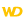WINDEV, WEBDEV AND WINDEV MOBILEONLINE HELPVersion: 15161718192021222324
 Home |Sign in | EnglishHelpWLanguageWLanguage functionsControls, pages and windowsDrawing functions (Image controls and variables)Object syntaxFunctions for drawing on controls (object syntax)DrawArcPresentationExampleCoordinates of the arcDrawing a solid figureRelated examplesSee alsoSaveICOAlphaBlendBackgroundChangeModeCopyDominantImageColorDrawArcDrawBorderDrawChordDrawCircleDrawLineDrawPointDrawPolygonDrawPolylineDrawRectangleDrawRoundedRectangleDrawSliceDrawTextDrawTextRTFEndDrawingFillFontHorizontalSymmetryInvertColorModifyHSLModifyHueModifyLightnessModifySaturationPenPixelColorPixelOpacityResizeSaveBMPSaveGIFSaveJPEGSavePNGStartDrawingVerticalSymmetrySaveTIFFDrawing functions (object syntax)dRectangleDegrade_objetNew WINDEV, WEBDEV, WINDEV Mobile 24 feature!
• Coordinates of the arc
• Drawing a solid figure
WINDEVWEBDEVWINDEV MobileOthers<Image>.DrawArc (Function)
In french: <Image>.DessineArc
Draws a circle arc or an ellipse arc in an Image control.
The arc is drawn anticlockwise.
Example
// Draw an arc of circle in light yellow
IMG_MyDrawing.DrawArc(10, 10, 50, 50, 20, 20, 40, 50, LightYellow)
Syntax
<Image control>.DrawArc(<X1> , <Y1> , <X2> , <Y2> , <X3> , <Y3> , <X4> , <Y4> [, <Line color> [, <Line thickness>]])
<Image control>: Control name
Name of Image control to use.
<X1>: Integer
X coordinate (in pixels) of top left corner of the rectangle containing the circle.
<Y1>: Integer
Y coordinate (in pixels) of top left corner of the rectangle containing the circle.
<X2>: Integer
X coordinate (in pixels) of bottom right corner of the rectangle containing the circle.
<Y2>: Integer
Y coordinate (in pixels) of bottom right corner of the rectangle containing the circle.
<X3>: Integer
X coordinate (in pixels) of start point of the arc of circle.
<Y3>: Integer
Y coordinate (in pixels) of start point of the arc of circle.
<X4>: Integer
X coordinate (in pixels) of end point of the arc of circle.
<Y4>: Integer
Y coordinate (in pixels) of end point of the arc of circle.
<Line color>: Integer or constant (optional)
Color of the arc of circle. This color corresponds to:
• an RGB color (returned by RGB),
• an HSL color (returned by HSL),
• a preset color of WLanguage,
•Versions 22 and latera Color variable.New in version 22a Color variable.a Color variable.a Color variable.
If this parameter is not specified, the line color:
<Line thickness>: Optional integerVersions 22 and laterThickness (in pixels) of the arc of circle to draw. If this parameter is not specified, the value taken into account is:New in version 22Thickness (in pixels) of the arc of circle to draw. If this parameter is not specified, the value taken into account is:Thickness (in pixels) of the arc of circle to draw. If this parameter is not specified, the value taken into account is:
Remarks

Coordinates of the arc

If the start and end points are not located on the circle border (or on the ellipse border), the point taken into account corresponds to the intersection between the circle and the line that joins the specified point and the center of rectangle (which means the center of the circle).The origin used to calculate the coordinates corresponds to the top left corner of Image control ((0,0) coordinates).

Drawing a solid figure

To draw a solid figure (a full arc), use <Image variable>.DrawChord or <Image variable>.DrawSlice.
Related Examples:Unit examples (WINDEV): The drawing functions [ + ] Using the main drawing functions of WINDEV to:- Initialize an Image control for drawing- Draw simple shapes- Write a text into a drawing- Change the color in a drawing
Component : wd240pnt.dll
Minimum version required
• Version 24